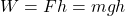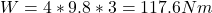## How much work is required to lift a 4kg object 3m high?

Question

How much work is required to lift a 4kg object 3m high?

in progress 0
2 months 2021-07-31T15:38:44+00:00 1 Answers 2 views 0

To lift the object it takes 117.6 N m of work.

Explanation:

Given data:

m = mass of the object = 4 kg

h = high = 3 m

Question: How much work, W = ?

The work:Here

g = gravity = 9.8 m/s²